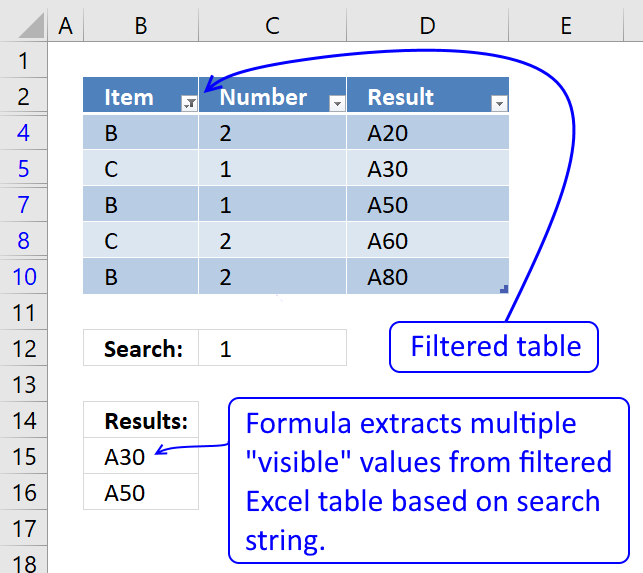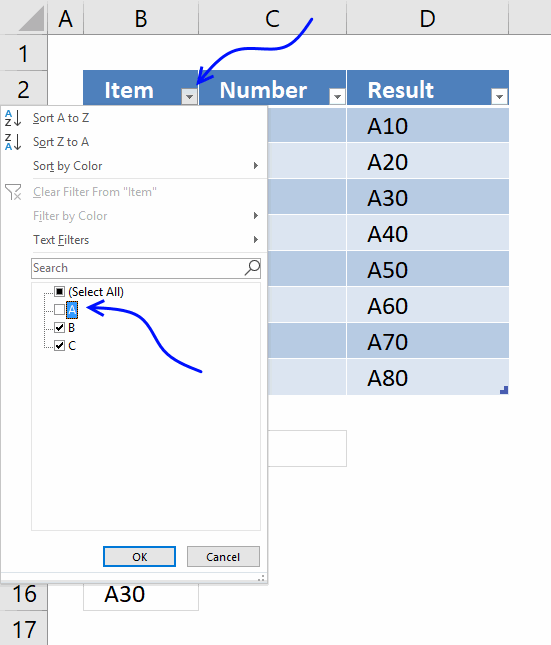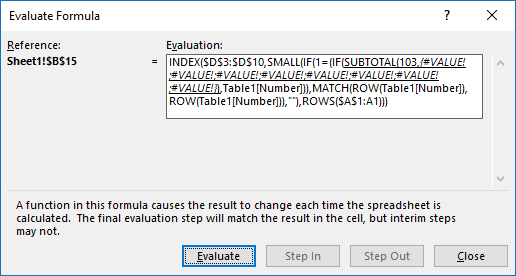Author: Oscar Cronquist Article last updated on January 29, 2019This post describes how to search filtered values in an Excel defined Table using a condition given in cell 12 and return multiple values. Some rows are hidden because of Excel Table filters, I am not using the VLOOKUP function in this formula.

Array Formula in cell B15:

=INDEX(Table1[Result], SMALL(IF(\$C\$12=(IF(SUBTOTAL(103, OFFSET(Table1[Number], MATCH(ROW(Table1[Number]), ROW(Table1[Number]))-1, 0, 1)), Table1[Number])), MATCH(ROW(Table1[Number]), ROW(Table1[Number])), ""), ROWS(\$A\$1:A1)))

#### How to create an array formula

1. Copy (Ctrl + c) and paste (Ctrl + v) array formula into formula bar.2. Press and hold Ctrl + Shift.
3. Press Enter once.
4. Release all keys.

#### Filter table

Let's remove all A's from item column. Press with left mouse button on black arrow near Item header. Deselect A. Press with left mouse button on OK.### Explaining formula in cell B15

#### Step 1 - Create array

This step is necessary in order to be able to use the SUBTOTAL function in the next step. The ROW function returns row numbers based on a cell reference.

The MATCH function converts the row number array to an array that starts with 1.

OFFSET(Table1[Number], MATCH(ROW(Table1[Number]), ROW(Table1[Number]))-1, 0, 1)

becomes

OFFSET(Table1[Number], MATCH({3;4;5;6;7;8;9;10}, {3;4;5;6;7;8;9;10})-1, 0, 1)

becomes

OFFSET(Table1[Number], {1;2;3;4;5;6;7;8} - 1, 0, 1)

becomes

OFFSET(Table1[Number], {0;1;2;3;4;5;6;7}, 0, 1)

becomes

OFFSET({2; 1; 2; 1; 2; 1; 2}, {0;1;2;3;4;5;6;7}, 0, 1)

and returns

{2; 1; 2; 1; 2; 1; 2}.

Note, the OFFSET function is volatile meaning it is recalculated each time the workbook is recalculated. Extensive use may slow down your workbook considerably.

#### Step 2 - Which values are shown in the Filtered Excel table

The SUBTOTAL(103, array) counts the number of values that are not empty.

SUBTOTAL(103, OFFSET(Table1[Number], MATCH(ROW(Table1[Number]), ROW(Table1[Number]))-1, 0, 1))

becomes

SUBTOTAL(103, {2; 1; 2; 1; 2; 1; 2})

and returns

{0; 1; 1; 0; 1; 1; 0; 1}

This array may look weird, the array in the SUBTOTAL function has 7 values and the result array has 8 values, how is it possible? This is, however, what you get if you convert the functions to constants by selecting them using and then pressing F9.

If you examine the formula using the "Evaluate Formula" tool you get the following array: {#VALUE!; #VALUE!; #VALUE!; #VALUE!; #VALUE!; #VALUE!; #VALUE!; #VALUE!}Even more confusing. This is what I think is going on. The OFFSET function actually returns an array of arrays, like this { {2}; {1}; {2}; {1}; {2}; {1}; {2} }

Then the SUBTOTAL function returns an array that indicates if the value is shown in the filtered table or not. 0 (zero) means not shown and 1 is visible.

{0; 1; 1; 0; 1; 1; 0; 1} meaning row 3, 6 and 9 are hidden in the filtered table.

#### Step 3 - Convert array to actual values

The IF function has three arguments, the first one must be a logical expression. If the expression evaluates to TRUE then one thing happens (argument 2) and if FALSE another thing happens (argument 3).

IF(SUBTOTAL(103, OFFSET(Table1[Number], MATCH(ROW(Table1[Number]), ROW(Table1[Number]))-1, 0, 1)), Table1[Number])

becomes

IF({0; 1; 1; 0; 1; 1; 0; 1}, Table1[Number])

and returns

{FALSE; 2; 1; FALSE; 1; 2; FALSE; 2}

#### Step 4 - Match values to condition specified in cell C12

IF(\$C\$12=(IF(SUBTOTAL(103, OFFSET(Table1[Number], MATCH(ROW(Table1[Number]), ROW(Table1[Number]))-1, 0, 1)), Table1[Number])), MATCH(ROW(Table1[Number]), ROW(Table1[Number])), "")

becomes

IF({FALSE; 2; 1; FALSE; 1; 2; FALSE; 2}, MATCH(ROW(Table1[Number]), ROW(Table1[Number])), "")

becomes

IF({FALSE; 2; 1; FALSE; 1; 2; FALSE; 2}, {1;2;3;4;5;6;7;8}, "")

and returns

{""; ""; 3; ""; 5; ""; ""; ""}

#### Step 5 - Extract k-th smallest row number

To be able to return a new value in a cell each I use the SMALL function to extract row numbers from smallest to largest.

The ROWS function keeps track of the numbers based on an expanding cell reference. It will expand as the formula is copied to the cells below.

SMALL(IF(\$C\$12=(IF(SUBTOTAL(103, OFFSET(Table1[Number], MATCH(ROW(Table1[Number]), ROW(Table1[Number]))-1, 0, 1)), Table1[Number])), MATCH(ROW(Table1[Number]), ROW(Table1[Number])), ""), ROWS(\$A\$1:A1))

becomes

SMALL({""; ""; 3; ""; 5; ""; ""; ""}, ROWS(\$A\$1:A1))

becomes

SMALL({""; ""; 3; ""; 5; ""; ""; ""}, 1)

and returns 3.

#### Step 6 - Return value based on row number

The INDEX function returns a value based on a cell reference and column/row numbers.

INDEX(Table1[Result], SMALL(IF(\$C\$12=(IF(SUBTOTAL(103, OFFSET(Table1[Number], MATCH(ROW(Table1[Number]), ROW(Table1[Number]))-1, 0, 1)), Table1[Number])), MATCH(ROW(Table1[Number]), ROW(Table1[Number])), ""), ROWS(\$A\$1:A1)))

becomes

INDEX(Table1[Result], 3)

becomes

INDEX({"A10"; "A20"; "A30"; "A40"; "A50"; "A60"; "A70"; "A80"}, 3)

and returns A30 in cell B15.

### Get the Excel filevlookup-a-filtered-table-and-return-multiple-values.xlsx